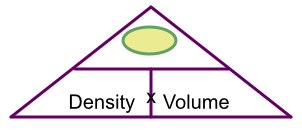Density

## Density

Density is the amount of mass in a volume. The more dense a substance is, the heavier it is for the same amount of volume.

The formula for density is:

text(density) = frac(text(mass))(text(volume))

Density is measured in g/cm3 or kg/m3.

## Example 1

A cuboid of lead, 3cm by 5cm by 2cm, has density of 11.34 g/cm3. What mass is the cuboid?

Volume = 3 x 5 x 2 = 30cm3.Cover up mass: left with density x volume

30 x 11.34 = 340.2g